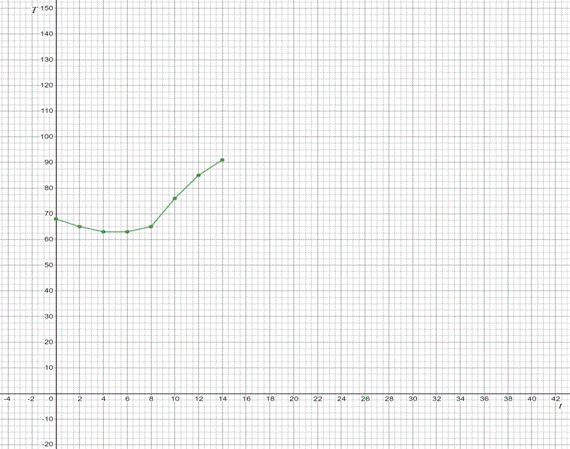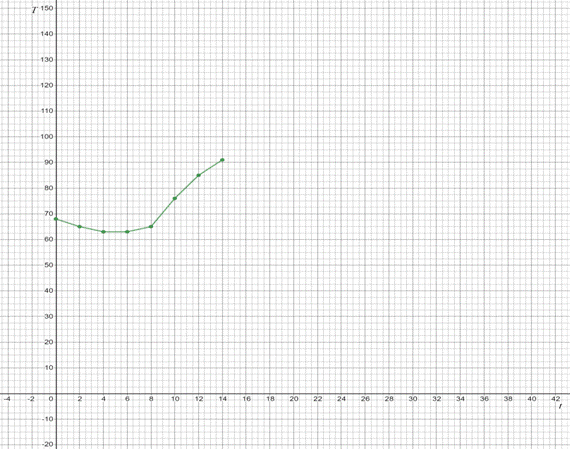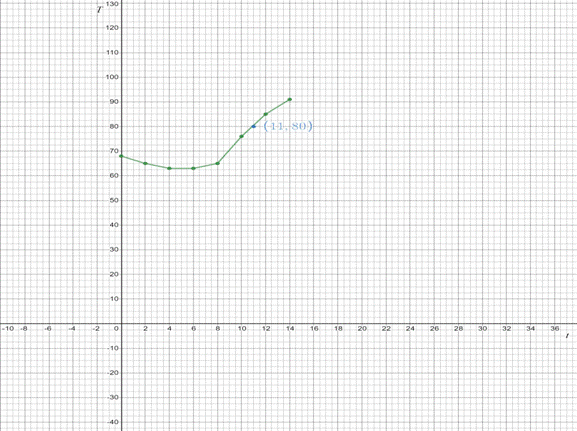# To graph : The temperature reading ( T ) as a function of ( t ) where the given data is as below, t 0 2 4 6 8 10 12 14 T 68 65 63 63 65 76 85 91### Single Variable Calculus: Concepts...

4th Edition
James Stewart
Publisher: Cengage Learning
ISBN: 9781337687805### Single Variable Calculus: Concepts...

4th Edition
James Stewart
Publisher: Cengage Learning
ISBN: 9781337687805

#### Solutions

Chapter 1.1, Problem 22E

a.

To determine

## To graph: The temperature reading (T) as a function of (t) where the given data is as below,t02468101214T6865636365768591

Expert Solution

### Explanation of Solution

Given information:

It is given that Temperature readings (in °F) were recorded every two hours from midnight to 2:00 PM in Baltimore on September 26, 2007. The time was measured in hours from midnight as shown in table below.

 t 0 2 4 6 8 10 12 14 T 68 65 63 63 65 76 85 91

Graph:

The graph of T vs t can be drawn by using t as x-axis and T as y-axis. The obtained graphs is as like this,Interpretation:

The obtained graph shows that first temperature decrease till 8 a.m. and then increases till 14:00 that means till 2 P.M.

b.

To determine

### To calculate: The temperature at 11:00 a.m. using the given graph.Expert Solution

The temperature at 11:00 a.m. is 80°F in the given graph.

### Explanation of Solution

Given information:

It is given that Temperature readings (in °F) were recorded every two hours from midnight to 2:00 PM in Baltimore on September 26, 2007. The time was measured in hours from midnight as shown in table below.

 t 0 2 4 6 8 10 12 14 T 68 65 63 63 65 76 85 91

Calculation:

Consider the given graph, the time 11:00 a.m. lies in between 10 to 12 on the x-axis. Now, take the corresponding value of y with respect to x as 11, it is find out to be 80 on this graph.Therefore, the temperature at 11:00 a.m. is 80°F in the given graph.

### Have a homework question?

Subscribe to bartleby learn! Ask subject matter experts 30 homework questions each month. Plus, you’ll have access to millions of step-by-step textbook answers!